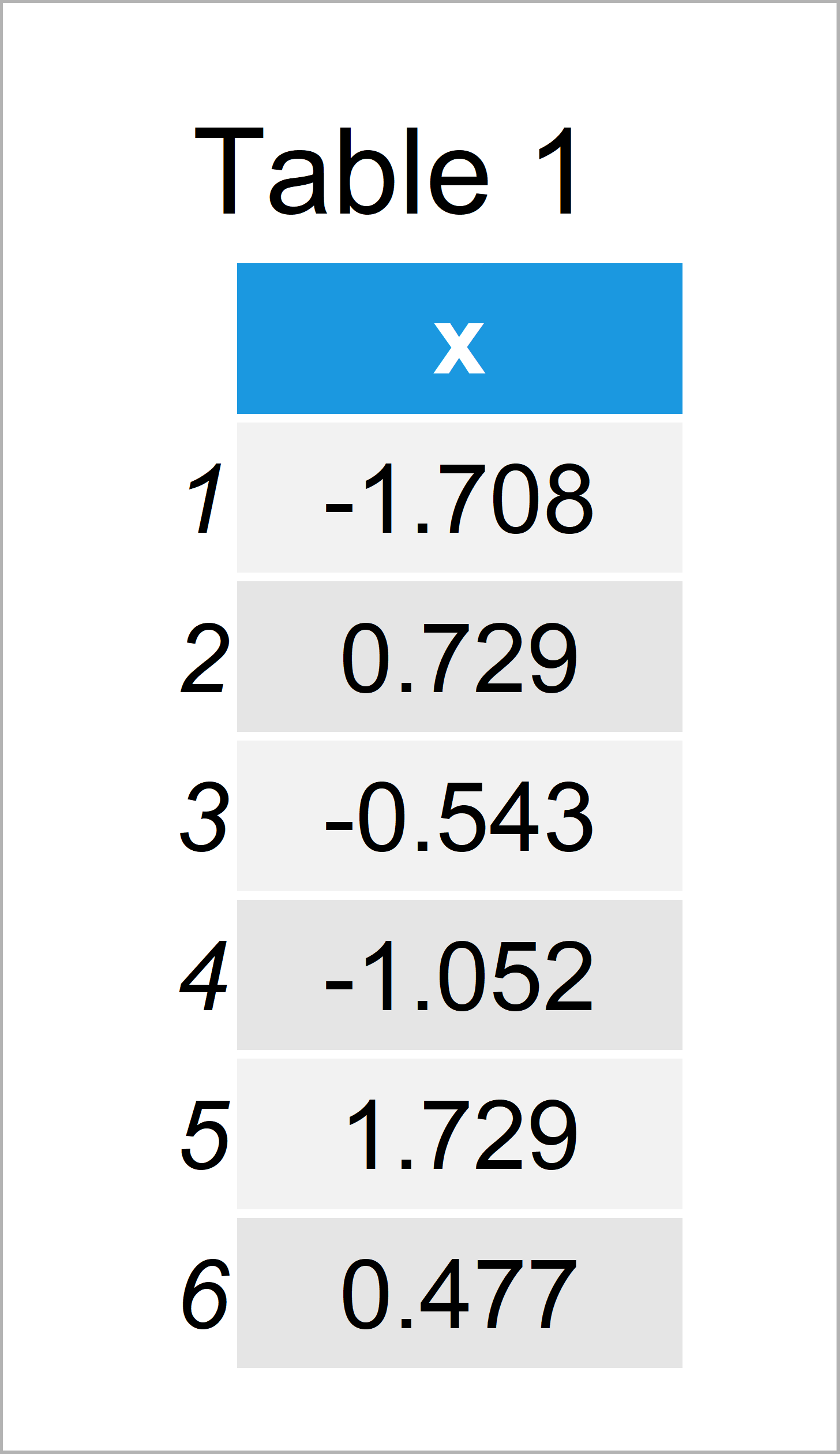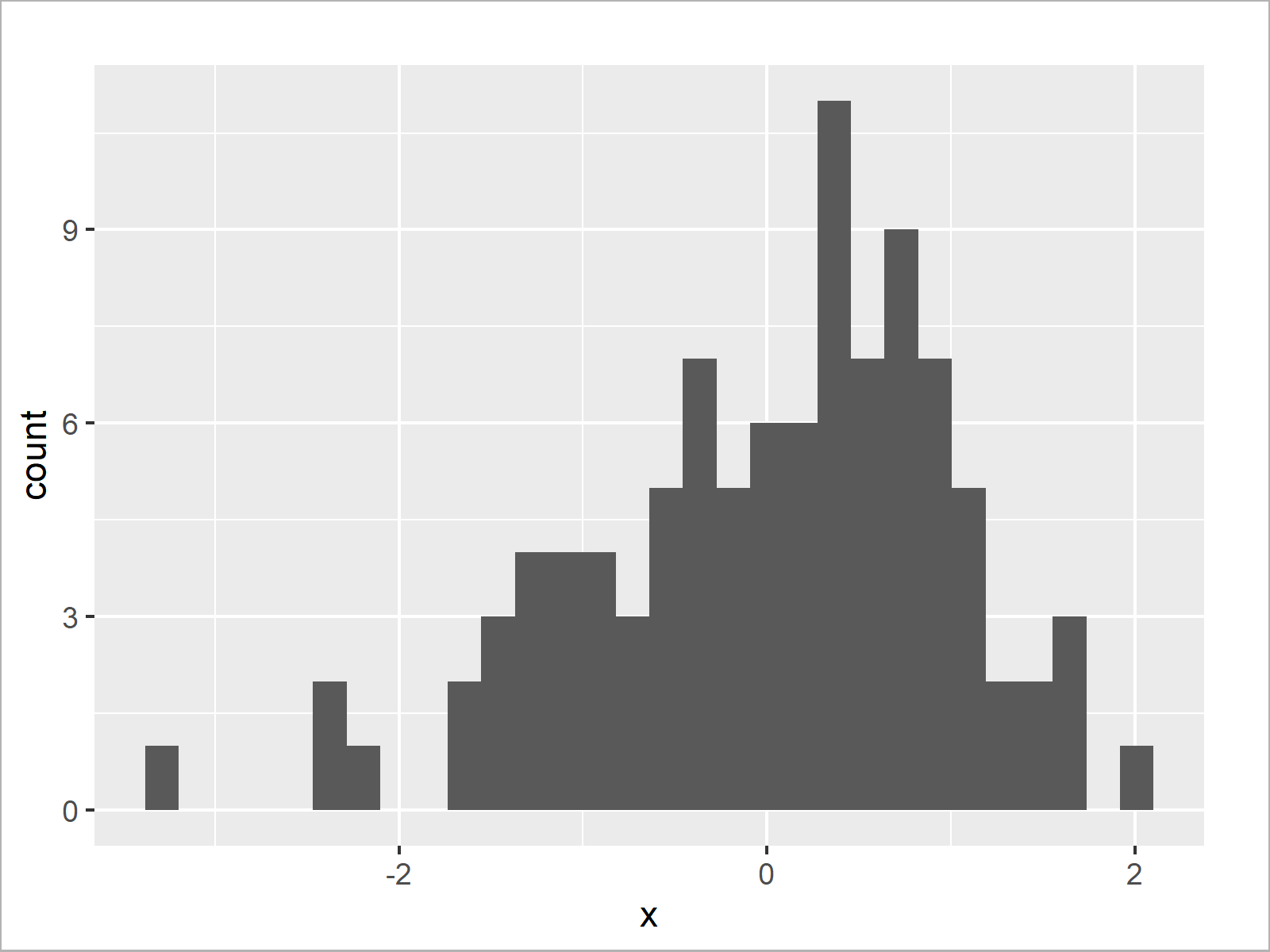# Set Number of Bins for Histogram in R (2 Examples)

In this article you’ll learn how to change the width of bins of a histogram in the R programming language.

The content of the tutorial is structured as follows:

Let’s dive right in!

## Creation of Exemplifying Data

First of all, we’ll need to create some data that we can use in the example syntax below:

```set.seed(8273456)                 # Create example data
data <- data.frame(x = rnorm(100))Table 1 illustrates the RStudio console output and shows the first six data points of our example data: Furthermore, you can see that our example data has only one column called “x”.

## Example 1: Modify Bins of Base R Histogram

Example 1 shows how to change the width of bins in a Base R histogram.

First, let’s create a histogram with default bin intervals:

`hist(data\$x)                      # Base R histogram with default bins`In Figure 1 you can see that we have created a Base R histogram with default bin width.

Now, we can use the breaks argument of the hist function to increase or decrease the width of our bars. In this example, we use a number of 100 bins:

`hist(data\$x, breaks = 100)        # Base R histogram with manual bins`In Figure 2 it is shown that we have managed to create a Base R histogram with thinner bars.

## Example 2: Modify Bins of ggplot2 Histogram

In Example 1, I have explained how to modify the bin width of a Base R histogram.

This example illustrates how to change the bar size of histograms using the ggplot2 package.

First, we need to install and load the ggplot2 package:

```install.packages("ggplot2")       # Install & load ggplot2 package
library("ggplot2")```

Now, we can use the ggplot and geom_histogram functions to create a ggplot2 histogram with default bin sizes:

```ggplot(data, aes(x)) +            # ggplot2 histogram with default bins
geom_histogram()```After running the previous R code the ggplot2 histogram with default bins shown in Figure 3 has been drawn.

Next, we can use the bins argument within the geom_histogram command to replace the default bin intervals:

```ggplot(data, aes(x)) +            # ggplot2 histogram with manual bins
geom_histogram(bins = 100)```Figure 4 shows the output of the previous syntax – A ggplot2 histogram with more bars.

## Video, Further Resources & Summary

Some time ago I have published a video instruction on my YouTube channel, which illustrates the R codes of this article. Please find the video below.

Please accept YouTube cookies to play this video. By accepting you will be accessing content from YouTube, a service provided by an external third party.If you accept this notice, your choice will be saved and the page will refresh.

In addition, you might read the other tutorials on my website. Some articles are shown below.

This article has shown how to set the number of bins in a histogram in the R programming language. Don’t hesitate to let me know in the comments section, if you have additional questions.

Subscribe to the Statistics Globe Newsletter